# convulution

23 de Mar de 2023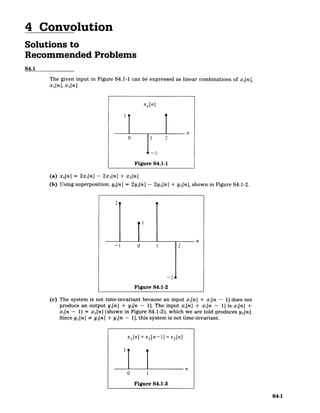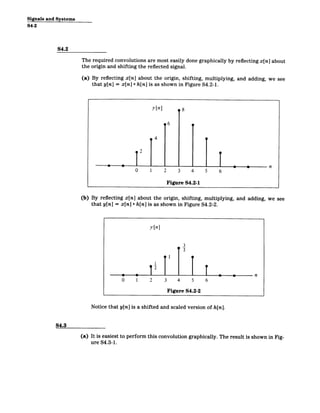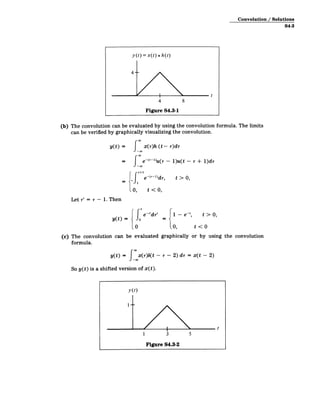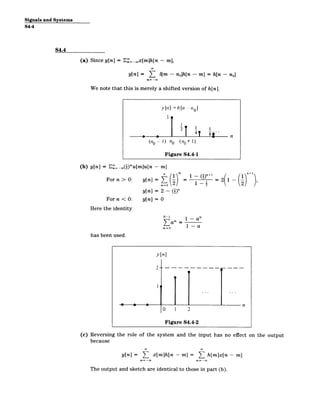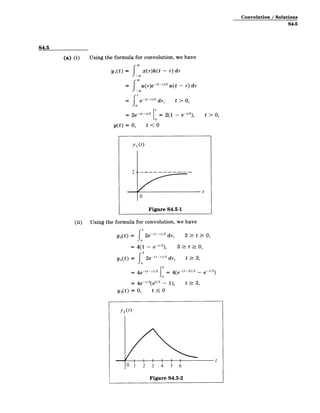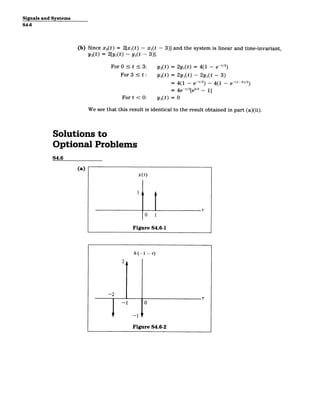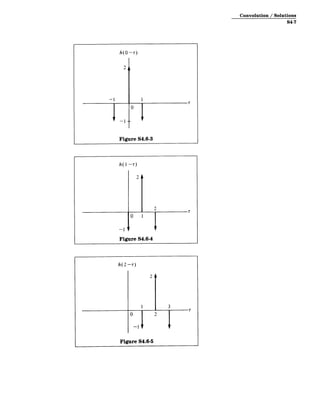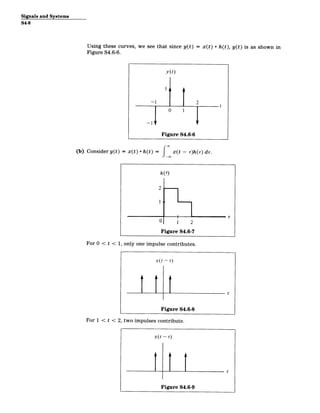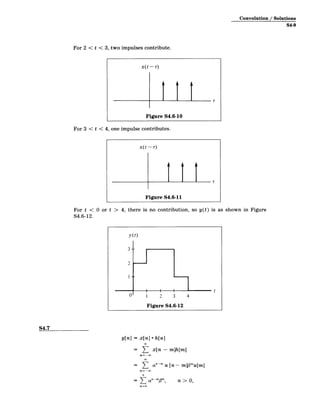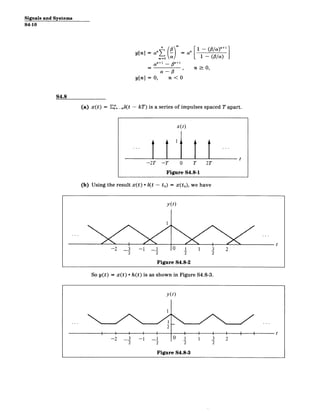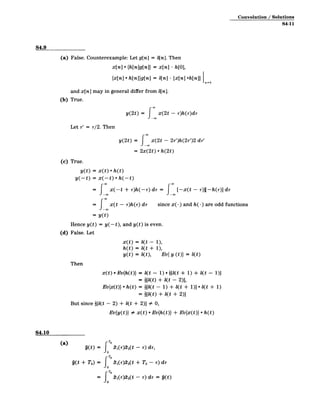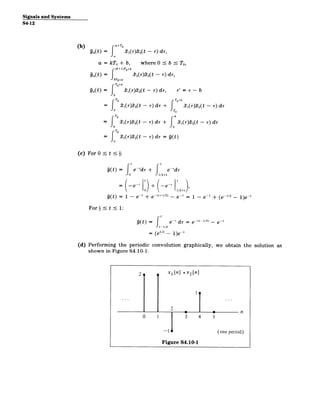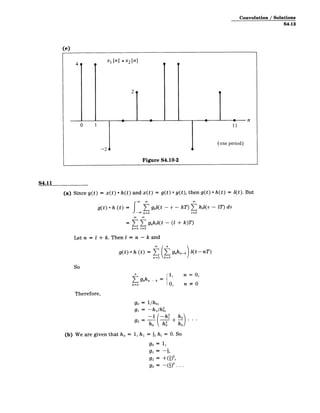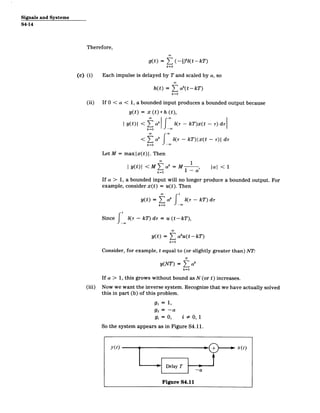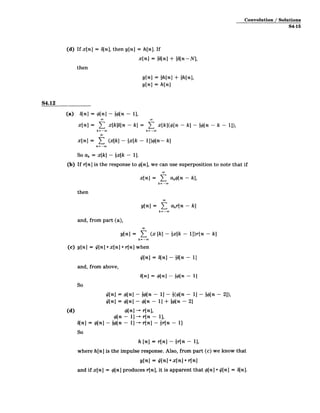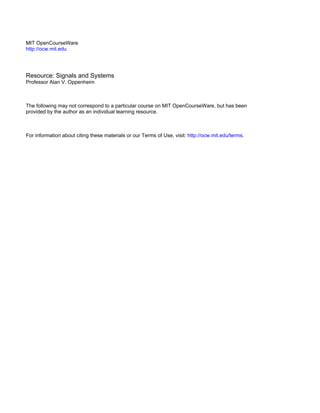Próximo SlideShare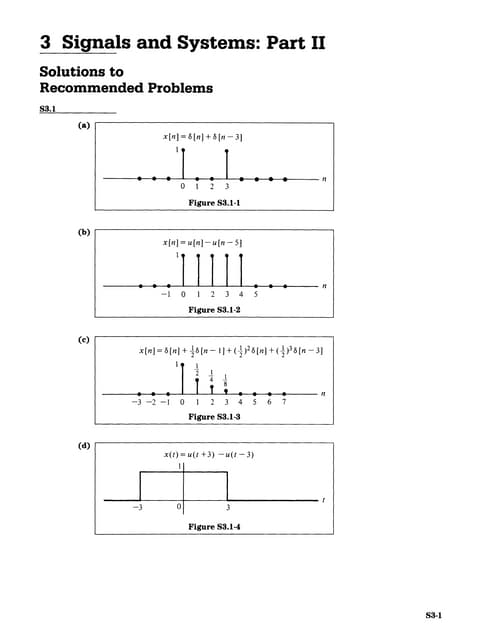Signals and Systems part 2 solutions
Cargando en ... 3
1 de 16

### convulution

1. 4 Convolution Solutions to Recommended Problems S4.1 The given input in Figure S4.1-1 can be expressed as linear combinations of xi[n], x 2[n], X3[n]. x,[n] 0 2 Figure S4.1-1 (a) x 4[n] = 2x 1 [n] - 2x 2[n] + x3[n] (b) Using superposition, y 4[n] = 2yi[n] - 2y 2[n] + y3 [n], shown in Figure S4.1-2. -1 0 1 Figure S4.1-2 (c) The system is not time-invariant because an input xi[n] + xi[n - 1] does not produce an output yi[n] + yi[n - 1]. The input x,[n] + xi[n - 11 is xi[n] + xi[n - 1] = x2[n] (shown in Figure S4.1-3), which we are told produces y 2[n]. Since y 2[n] # yi[n] + yi[n - 1], this system is not time-invariant. x 1 [n] +x 1 [n-1] =x2[n] n 0 1 Figure S4.1-3 S4-1
2. Signals and Systems S4-2 S4.2 The required convolutions are most easily done graphically by reflecting x[n] about the origin and shifting the reflected signal. (a) By reflecting x[n] about the origin, shifting, multiplying, and adding, we see that y[n] = x[n] * h[n] is as shown in Figure S4.2-1. (b) By reflecting x[n] about the origin, shifting, multiplying, and adding, we see that y[n] = x[n] * h[n] is as shown in Figure S4.2-2. y[n] 3 2 0 1 2 3 4 5 6 Figure S4.2-2 Notice that y[n] is a shifted and scaled version of h[n]. S4.3 (a) It is easiest to perform this convolution graphically. The result is shown in Fig­ ure S4.3-1.
3. Convolution / Solutions S4-3 y(t) = x(t) * h(t) 4-­ | t 4 8 Figure S4.3-1 (b) The convolution can be evaluated by using the convolution formula. The limits can be verified by graphically visualizing the convolution. y(t) = 7x(r)h (t - r)dr = e-'-Ou(r - 1)u(t - r + 1)dr 1 t+ e (- dr, t > 0, -0, t < 0, Let r' = T - 1. Then y( ) e- d r -e t > 0, 0 0 , t < 0 (c) The convolution can be evaluated graphically or by using the convolution formula. y(t) = x(r)6(t - , - 2) dr = x(t - 2) So y(t) is a shifted version of x(t). y(t) It - I / , t 1 3 5 Figure S4.3-2
4. Signals and Systems S4-4 S4.4 (a) Since y[n] = E=-oox[m]h[n - m], y[n] = 6 b[m - no]h[n - m] = h[n - no] m= -oO We note that this is merely a shifted version of h[n]. y [n] = h1[12­ I ae|41 8 n (n 1) no (n1+ 1) Figure S4.4-1 (b) y[n] = E =_c(!)'u[m]u[n ­ m] For n > 0: y[n] = 1 + = 2( 1 y[n] = 2 - (i)" Forn < 0: y[n] = 0 Here the identity N-i N _ T am Mr=O 1 ­ a has been used. y[n] 2--­ 140 0 1 2 Figure S4.4-2 (c) Reversing the role of the system and the input has no effect because y[n] = E x[m]h[n ­ m] = L h[m]x[n ­ m] m=-oo The output and sketch are identical to those in part (b). , on the output
5. Convolution / Solutions S4-5 S4.5 (a) (i) Using the formula for convolution, we have y 1 (t) = x(r)h(t - r) dr = r(-)-2u(t - r) dr t = e-( - 2 dr, t > 0, 2e 10 = 2(1 e t > 0, y(t) = 0, t < 0 y1 (t) - 2 - t 0 Figure S4.5-1 (ii) Using the formula for convolution, we have y2(t) = 2e-(t-r)/2 dr, 3 t>- 0, y2 (t) =4(1 - e-t/2), = { 2e-(-­ /2 d-, 3 t : 0, t >_3, 3 S4e (t-r)/2 0 4(e -(t-3)/2 _ e-t/2 0 = 4e- t/2 (e'/ 2 - 1), t 3, y 2(t) = 0, t 0 y 2 (t) 0 1 2 3 4 5 6 Figure S4.5-2
6. Signals and Systems S4-6 (b) Since x 2(t) = 2[xl(t) - xl(t - 3)] and the system is linear and time-invariant, y2(t) = 2[yi(t) - y1(t - 3)]. For 0 s t s 3: y2(t) = 2yi(t) = 4(1 - e-'/2) For 3 t y 2(t) = 2y,(t) - 2yi(t - 3) = 4(1 - e-1/2 4(1 - e- t -3)2) = 4e- t/2 e 3 / 2 _ 1 Fort< 0: y2(t) = 0 We see that this result is identical to the result obtained in part (a)(ii). Solutions to Optional Problems S4.6 (a) x(T) 1 T 0 1 Figure S4.6-1 h(-1 -r) 2, -2 T -- 1 0 --1 ' Figure S4.6-2
7. Convolution / Solutions S4-7 h(0-r) Figure S4.6-3 h(1-- ) Figure S4.6-4
8. Signals and Systems S4-8 Using these curves, we Figure S4.6-6. see that since y(t) = x(t) * h(t), y(t) is as shown in (b) Consider y(t) = x(t) * h(t) = 1 -- --- f0x(t - r)h(r)dr. y(t) 1 W 2 0 1 4 Figure S4.6-6 t h(r) 2 1 For 0 < t < 0 1 2 Figure S4.6-7 1, only one impulse contributes. x(t -r) For 1 < Figure S4.6-8 t < 2, two impulses contribute. x(t ­ ) Figure S4.6-9
9. Convolution / Solutions S4-9 For 2 < t < 3, two impulses contribute. x(t- r) Figure S4.6-10 For 3 < t < 4, one impulse contributes. t) x(t­ Figure S4.6-11 For t < 0 or t > 4, there is no contribution, so y(t) is as shown in Figure S4.6-12. y(t) 3 -­ 2 I-­ U' 1 2 3 4 Figure S4.6-12 S4.7 y[n] = x[n] * h[n] = 1 x[n - m]h[m] nO =- - n 0, anmm, > M=0
10. Signals and Systems S4-10 y[n] = a" = a" L (la) a n+1 _ n+1 a - # n > 0, y[n] = 0, n < 0 S4.8 (a) x(t) = E_= - kT) is a series of impulses spaced T apart. x(t) t -2T -T 0 T 2T Figure S4.8-1 (b) Using the result x(t) *(t - to) = x(to), we have y(t) .. t -2 3 -1 3 3 0 1 1 2 2 2 2 2 Figure S4.8-2 So y(t) = x(t) * h(t) is as shown in Figure S4.8-3. y(t) 2 -2 -3 -1 -j 0 1 1 3 2 22 2 2 Figure S4.8-3
11. Convolution / Solutions S4-11 S4.9 (a) False. Counterexample: Let g[n] = b[n]. Then x[n] * {h[n]g[n]} = x[n] h, {x[n]* h[n]}g[n] = b[n] [x[n] *h[n]] n=0 and x[n] may in general differ from b[n]. (b) True. y(2t) = fx(2t - r)h(r)dr Let r' = T/ 2 . Then y(2t) = f x(2t - 2r')h(2r')2dr' = 2x(2t)* h(2t) (c) True. y(t) = x(t) * h(t) y(- t) = x(-t) * h(-t) -f x(-t + r)h(-r)dr = f [-x(t - r)][-h(r)] dT =f x(t - r)h(r)dr since x(-) and h(-) are odd fu ictions - y(t) Hence y( t) = y(-t), and y(t) is even. (d) False. Let x(t) = b(t - 1) h(t) = b(t + 1) y(t) = b(t), Ev{ y (t)} = 6(t) Then x(t) *Ev{h(t)} =b(t - 1) *i[b(t + 1) + b(t - 1)] = i[b(t) + b(t - 2)], Ev{x(t)} * h(t) =1[6t - 1) + b(t + 1)] * b(t + 1) = 1[b(t) + b(t + 2)] But since 1[6(t - 2) + b(t + 2)] # 0, Ev{y(t)} # x(t) *Ev{h(t)} + Ev{x(t)} *h(t) S4.10 (a) 9(t) = Jro TO21 (r)22(t - r) dr, O D(t + T0) = J 21 (T)- 2(t + To - r) d-r = TO 1 (r) 2 (t - r)dr = (t)
12. Signals and Systems S4-12 (b) 9a(t) = T a+TO 2 1(i)2 2(t - -) dr, a 9a(t) = = kTo + b, where 0 (k+1)T0+b 2 1(r)A 2(t - i) kT0+b TO+b b - dr, To, Pa(t) = fb 2 1 (i)± 2 (t - r) di, i' = i - b FTo T70+b = T1()- t - r) dr + 1&)2(t - r) di 2 b TO Tb ()A - r) d1 2- ) di = ) T)TO = 21 )12( t T ) dr =q t ) t(-r ­ (c) For 0 s t - I ft e- I 9(t) = di + Ti±e-'di 0e + e/2+t1/2+1 =(-e-' t + (-e-T 0 11/2+t) (t) = 1 - e~' + e-(*±1/2) - e-1 = 1 - e-1 + (e 1/ 2 - 1)e- For s t < 1: = ft t(t) e-' di = e- ( 1/2) - e­ - 1/2 )e (d) Performing the periodic convolution graphically, we obtain the solution as shown in Figure S4.10-1. 2 X1[n] *x2[n] 19 0 1 3 4 5 -16 (one period) Figure S4.10-1
13. Convolution / Solutions S4-13 (e) S4.11 (a) Since y(t) = x(t) *h (t) and x(t) = g(t) *y(t), then g(t) *h(t) = 6(t). But g(t )*h (t) = grob t - kT hboTr - lT d, wk=0 1=0 = T gkhlo(t - (1+ k)T) k=O 1=0 Let n = 1 + k. Then = n - k and g(t)* h (t) = Ygkhn t - n n=0 k=0 n F, n = 0, k=0 n O Therefore, go = 1/h 0 , gi = -- hi/ho, 1 (-hl h2) 2 o ho ho (b) We are given that h0 = 1, hi = I, hi = 0. So = 1, g1 = -i) g2=+(1)2, g2 = -()
14. Signals and Systems S4-14 Therefore, g(t) = ( (-) k=O (tkT) (c) (i) Each impulse is delayed by T and scaled by a, so h(t) = k=O (t -kT ) (ii) If 0 < a < 1, a bounded input produces a bounded output because y(t) = x (t)* h (t), | y(t)| < Zak k=0 < a k=O f 6(r ­ kT)x(t - r) di -w _(T - kT)Ix(t - T)I dr -w Let M = maxlx(t)|. Then 1 I y(t) < M ak= M , al < 1 k=O If a > 1, a bounded input will no longer produce a bounded output. For example, consider x(t) = u(t). Then 00 t yt) = Ta f 6(,r - kT) dr k=O -w Since f 6(r - kD di = u (t-kT ), y(t) = ( aku(t-kT) k=O Consider, for example, t equal to (or slightly greater than) NT: N y(NT) = Z ak k=O (iii) If a > 1, this grows without bound as N (or t) increases. Now we want the inverse system. Recognize that we have actually solved this in part (b) of this problem. gi = 1, g2 = -a gi = 0, i # 0, 1 So the system appears as in Figure S4.11. y(t) _0_ x(t) Delay T Figure S4.11
15. Convolution / Solutions S4-15 (d) If x[n] = 6[n], then y[n] = h[n]. If x[n] = go[n] + iS[n-N], then y[n] = -h[n] + -h[n], y[n] = h[n] S4.12 (a) b[n] = #[n] - -4[n - 1], x[n] = ( x[k][n - k] = ( x[k]Q([n - k] - -p[n - k - 1]), k=-- k=--w x[n] = E (x[k] - ix[k - 1])4[n- k] k= -w So ak = x[k] - lx[k - 1]. (b) If r[n] is the response to #[n], we can use superposition to note that if x[n] = ( akp[n - k], k=­ then y[n] = Z akr[n - k] k= -w and, from part (a), y[n] = ( (x [k] - fx[k - 1])r[n - k] k= ­ (c) y[n] = i/[n] *x[n] * r[n] when [n] = b[n] - in- 1] and, from above, 3[n] 4[n] - -[- 1] So /[n] = #[n] - -#[n - 11 - 1(#[n - 11 - -\$[n - 2]), 0[n] = *[n] - *[n - 1] + {1[n - 2] (d) 4[n] - r[n], #[n - 1] - r[n - 1], b[n] = 4[n] - -1[n- 1] - r[n] - fr[n -1] So h[n] = r[n] - tr[n -1], where h[n] is the impulse response. Also, from part (c) we know that y[n] = Q[n] *x[n] *r[n] and if x[n] = #[n] produces r[n], it is apparent that #[n] * 4[n] = 6[n].
16. MIT OpenCourseWare http://ocw.mit.edu Resource: Signals and Systems Professor Alan V. Oppenheim The following may not correspond to a particular course on MIT OpenCourseWare, but has been provided by the author as an individual learning resource. For information about citing these materials or our Terms of Use, visit: http://ocw.mit.edu/terms.